# GRE Subject Test: Chemistry : HNMR and CNMR Spectroscopy

## Example Questions

### Example Question #2 : Compound Identification

Which of the following most likely represents the H-NMR spectrum of the molecule shown below?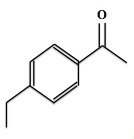Two triplets, two doublets, and one singlet

One quartet, two triplets, one doublet, and one singlet

One quartet, one triplet, two doublets, and one singlet

One quartet, one triplet, and three doublets

One quartet, one triplet, one doublet, and one singlet

One quartet, one triplet, two doublets, and one singlet

Explanation:

There are four total aromatic protons, consistent with two sets of identical pairs. This would result in two distinct aromatic signals, each having a doublet and each integrating two protons.

The methyl protons next to the ketone would be deshielded by the electron withdrawing ketone group, resulting in a downfield shift. The signal would be a singlet, since there are no neighboring protons to the methyl group.

Finally, the ethyl group would have two signals, one for the two protons next to the aromatic ring (shifted downfield because of the aromatic ring), and one highly shielded peak corresponding to the terminal protons. The protons next to the aromatic ring will result in a quartet from the three neighboring hydrogens, while the terminal peak will be a triplet from the two neighboring hydrogens.

The final result is one quartet (ethyl), one triplet (ethyl-terminal), two doublets (aromatic), and one singlet (methyl).

### Example Question #1 : Hnmr And Cnmr Spectroscopy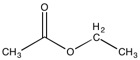Which of the following observations would most likely be seen when performing an H-NMR on 1-ethyl ethanoate (above)?

One singlet and one quartet

One doublet and one triplet

One singlet and two triplets

A singlet, a doublet, and a triplet

A singlet, a triplet, and a quartet

A singlet, a triplet, and a quartet

Explanation: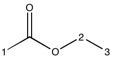Looking at the structure above, we can see that the molecule only contains three carbons bonded to protons. These carbons are labeled 1, 2 and 3.

An important concept in NMR questions is determining if two carbons on the same compound will have protons split identically, and thus indistinguishable in an NMR (i.e. will those two carbons represent two individual peaks or one large peak?). In this case, C1 and C3 are clearly distinguishable from C2, since C1 and C3 are bonded to 3 hydrogens, while C2 is only bonded to two. Because C2 is adjacent to a three proton carbon, we know that the splitting pattern will display at least one quartet. This will narrow our answer choices down to two options.

Because C1 and C3 contain the same number of protons, we need to determine if they will represent one large peak, or two separate peaks. Looking at the compound, we can see that C3 is adjacent to a two-proton carbon in C2, while C1 is not adjacent to any proton-bonded carbons; therefore, we can expect that C1 will not be split by any protons, and will display a singlet, and C3 will be split by 2 protons, and will display a triplet.

As a final result, we would expect to see one singlet, one triplet and one quartet.

### Example Question #2 : How To Identify Compounds

All of the following molecules would exhibit two distinct singlets in a 1H-NMR spectrum except __________.

1,4-dimethylbenzene

methyl-tert-butyl ether

1,3,5-trimethylbenzene

1,2,4,5-tetramethylbenzene

Explanation:

2,4-hexadiyne has only one 1H-NMR signal, as the two terminal methyl groups are identical and will have the same chemical shift.

1,2,4,5-tetramethylbenzene has two singlets: one for the four methyl groups and one for the two aromatic protons. Likewise, 1,3,5-trimethylbenzene will have two singlets: one for the three methyl groups (nine hydrogens total) and one for the three aromatic protons, which are all identical.

Methyl tert-butyl ether also has two singlets, one corresponding to the tert-butyl methyl protons, and one corresponding to the methoxy protons.

Finally, 1,4-dimethylbenzene has two singlets, one for the methyl groups, and one for the four aromatic protons, which are all identical.

### Example Question #1 : Hnmr And Cnmr SpectroscopyObserve the underlined/bold hydrogen. In HNMR, how many spectral lines will that bolded hydrogen be split into?lines (doublet)lines (triplet)lines (multiplet)linesline (singlet)lines (multiplet)

Explanation: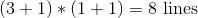Within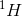NMR spectroscopy there are a couple important factors to understand, including the ppm shift (delta) and the splitting pattern. Here we are focusing on the splitting pattern for individual hydrogens. This is important for it lays the groundwork for understanding the patterns of peaks seen on large compound NMR’s.

When determining the splitting of any hydrogen you must use the n+1 rule. Before going into that rule we must understand two things, 1. Only nonequivalent hydrogens (protons) couple and 2. Usually they only couple to other hydrogens (protons) attached to adjacent carbons. Nonequivalent means the protons occupy their own unique spatial environment with different atoms surrounding them. Typically two hydrogens attached to the same carbon are equivalent (though this isn’t always the case and you must think about where the hydrogens are located in space and see whether they are adjacent to different or similar chemical groups).

The n+1 rule is performed as follows. n stands for the number of equivalent protons that are not equivalent to the proton of interest (the one we are trying to determine the splitting for). We multiply together each group of protons that are nonequivalent to the proton of interest. For example, lets say there are two groups of protons that are nonequivalent to the proton of interest, in group A there are 2 protons, and in group B there are 3 protons. We would use the n+1 rule for group A and get (2+1) = 3 and for group B get (3+1) = 4. We would then multiply these two numbers together to get the splitting for the proton of interest, thus there are 12 lines that the proton of interest would be split into. Proper use of this rule should allow you to get all NMR splitting questions correct (and elevate your understanding of why a certain NMR printout looks the way it does).

### Example Question #2 : Hnmr And Cnmr SpectroscopyObserve the underlined/bold hydrogen. In HNMR, how many spectral lines will that bolded hydrogen be split into?lines (doublet)lines (doublet of triplets)lines (septet)line (singlet)lines (doublet of doublets)lines (septet)

Explanation: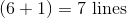WithinNMR spectroscopy there are a couple important factors to understand, including the ppm shift (delta) and the splitting pattern. Here we are focusing on the splitting pattern for individual hydrogens. This is important for it lays the groundwork for understanding the patterns of peaks seen on large compound NMR’s.

When determining the splitting of any hydrogen you must use the n+1 rule. Before going into that rule we must understand two things, 1. only nonequivalent hydrogens (protons) couple and 2. usually they only couple to other hydrogens (protons) attached to adjacent carbons. Nonequivalent means the protons occupy their own unique spatial environment with different atoms surrounding them. Typically two hydrogens attached to the same carbon are equivalent (though this isn’t always the case and you must think about where the hydrogens are located in space and see whether they are adjacent to different or similar chemical groups).

The n+1 rule is performed as follows. N stands for the number of equivalent protons that are not equivalent to the proton of interest (the one we are trying to determine the splitting for). We multiply together each group of protons that are nonequivalent to the proton of interest. For example, lets say there are two groups of protons that are nonequivalent to the proton of interest, in group A there are 2 protons, and in group B there are 3 protons. We would use the n+1 rule for group A and get (2+1) = 3 and for group B get (3+1) = 4. We would then multiply these two numbers together to get the splitting for the proton of interest, thus 3 x 4 = 12 lines that the proton of interest would be split into. Proper use of this rule should allow you to get all NMR splitting questions correct (and elevate your understanding of why a certain NMR printout looks the way it does).

### Example Question #1 : Hnmr And Cnmr SpectroscopyObserve the underlined/bold hydrogen. In HNMR, how many spectral lines will that bolded hydrogen be split into?lines (triplet)line (singlet)lines (multiplet)lines (multiplet)lineslines (multiplet)

Explanation: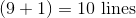WithinNMR spectroscopy there are a couple important factors to understand, including the ppm shift (Delta) and the splitting pattern. Here we are focusing on the splitting pattern for individual hydrogens. This is important for it lays the groundwork for understanding the patterns of peaks seen on large compound NMR’s.

When determining the splitting of any hydrogen you must use the n+1 rule. Before going into that rule we must understand two things, 1. only nonequivalent hydrogens (protons) couple and 2. usually they only couple to other hydrogens (protons) attached to adjacent carbons. Nonequivalent means the protons occupy their own unique spatial environment with different atoms surrounding them. Typically two hydrogens attached to the same carbon are equivalent (though this isn’t always the case and you must think about where the hydrogens are located in space and see whether they are adjacent to different or similar chemical groups).

The n+1 rule is performed as follows. N stands for the number of equivalent protons that are not equivalent to the proton of interest (the one we are trying to determine the splitting for). We multiply together each group of protons that are nonequivalent to the proton of interest. For example, lets say there are two groups of protons that are nonequivalent to the proton of interest, in group A there are 2 protons, and in group B there are 3 protons. We would use the n+1 rule for group A and get (2+1) = 3 and for group B get (3+1) = 4. We would then multiply these two numbers together to get the splitting for the proton of interest, thus 3 x 4 = 12 lines that the proton of interest would be split into. Proper use of this rule should allow you to get all NMR splitting questions correct (and elevate your understanding of why a certain NMR printout looks the way it does).

### Example Question #1 : Hnmr And Cnmr SpectroscopyObserve the underlined/bold hydrogen. In HNMR, how many spectral lines will that bolded hydrogen be split into?lines (triplet)lines (multiplet)line (singlet)lines (septet)lines (doublet)lines (doublet)

Explanation: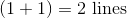Within 1H NMR spectroscopy there are a couple important factors to understand, including the ppm shift (delta) and the splitting pattern. Here we are focusing on the splitting pattern for individual hydrogens. This is important for it lays the groundwork for understanding the patterns of peaks seen on large compound NMR’s.

When determining the splitting of any hydrogen you must use the n+1 rule. Before going into that rule we must understand two things, 1. only nonequivalent hydrogens (protons) couple and 2. usually they only couple to other hydrogens (protons) attached to adjacent carbons. Nonequivalent means the protons occupy their own unique spatial environment with different atoms surrounding them. Typically two hydrogens attached to the same carbon are equivalent (though this isn’t always the case and you must think about where the hydrogens are located in space and see whether they are adjacent to different or similar chemical groups).

The n+1 rule is performed as follows. N stands for the number of equivalent protons that are not equivalent to the proton of interest (the one we are trying to determine the splitting for). We multiply together each group of protons that are nonequivalent to the proton of interest. For example, lets say there are two groups of protons that are nonequivalent to the proton of interest, in group A there are 2 protons, and in group B there are 3 protons. We would use the n+1 rule for group A and get (2+1) = 3 and for group B get (3+1) = 4. We would then multiply these two numbers together to get the splitting for the proton of interest, thus 3 x 4 = 12 lines that the proton of interest would be split into. Proper use of this rule should allow you to get all NMR splitting questions correct (and elevate your understanding of why a certain NMR printout looks the way it does).

### Example Question #1 : Hnmr And Cnmr Spectroscopy

Which of the following spectroscopic techniques provides the most information about an organic molecule's framework/structure?

Mass spectrometry

NMR spectroscopy

Melting point

IR spectroscopy

UV visible spectroscopy

NMR spectroscopy is most useful for determining the type of nuclei (most commonly studied nuclei are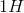and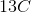) present and their relative locations within a molecule. H-NMR is most commonly used because it is practically present in all organic compounds. This technique is useful for a complete determination of the structure of organic compounds. IR spectroscopy is best for determining the functional groups of a molecule, however, it does not give information of the electric environment like NMR. Mass spectrometry is a technique used to determine the amount and mass of substances present in a sample. UV-visible spectroscopy is used to determine the amount of analyte present in a given sample - this method is best for transition metals and/or highly conjugated compounds. Melting point analysis gives information about the purity of a sample, pure substances tend to have higher melting points and more narrow ranges than impure samples.